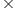Maths-
General
Easy

Question

# A cubical tank 50 cm in length and 36 cm in breadth contain water. A cube of x cm edge is dropped into it and fully immersed. If the rise in water level is 15 cm, solve for x. and hence find the T.S.A of the cubical tank

## 30 ,620040 , 620030, 540040, 5400Hint:

## The correct answer is: 30 ,6200

### We have given the dimensions of cubical tankLength l = 50cmBreadth b= 36cmThe dimensions of cube dropped areSide = x cmWe have to find the value of x and TSA of cubical tank.It is given that rise in water level is 15 cm , so it will be the height of the water in the cubical tankh = 15 cmTherefore the volume of the cube dropped will beVolume = lbhx3 = 503615= 27000As 27000 is a cube of 30x3 = (30)3therefore, x = 30The TSA of the cubical tank = 2[lb + bh + hl]= 2[(50)(36) + (36)(15) + (15)(50)l]= 2[1800 + 540 + 750]= 2= 6180Which is approximately equal to 6200.Therefore, the correct option is a) 30 , 6200.#### With Turito Foundation.#### Get an Expert Advice From Turito.